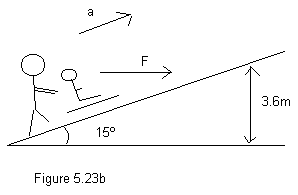# Calculating the Work done from an Incline with Friction Problem

Iconic
Problem:
A father pushes horizontally on his daughter's sled to move it up a snowy incline, as illustrated in the figure, with h = 3.6m and θ = 15°. The total mass of the sled and the girl is 35 kg and the coefficient of kinetic friction between the sled runners and the snow is 0.20. If the sled moves up the hill with a constant velocity, how much work is done by the father in moving it from the bottom to the top of the hill?Equations Used:

Forcenet Y components ƩFy= N-mgcosθ=0-(due to constant velocity)
So...
Normal Force Fn= mgcosθ
And since...
Friction Force Ff= (μ)kFn
So...
Friction Force Ff= (μ)k(mgcosθ)

Forcenet X components ƩFx= -mgsinθ-(μ)k(mgcosθ)

## The Attempt at a Solution

Before I go any further with solving this problem I need to know how to use the applied Force from the father in this problem. I'm 100% certain my ƩFx is incorrect and was hoping someone could explain how I should fix this.

Last edited: